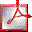# Bayesian Single-Case Methods

## Programs: SingleBayes.exe, DiffBayes.exe and DissocsBayes.exe

These programs for PCs accompany the paper: Crawford, J. R., & Garthwaite, P. H. (2007). Comparison of a single case to a control or normative sample in neuropsychology: Development of a Bayesian approach. Cognitive Neuropsychology, 24, 343-372.

The programs implement Bayesian inferential methods for use in single-case studies in which a patient is compared to a control sample. A convenient feature of these programs (and other programs available on these pages) is that they take summary statistics from the control population as inputs. For SingleBayes.exe the control mean, standard deviation and sample size are required. For programs examining differences between two tasks (DiffBayes.exe and DissocsBayes.exe) the inputs required are the means and SDs for the two tasks in controls, together with the correlation between tasks in controls and the sample n.

SingleBayes.exe uses Bayesian Monte Carlo methods to test if a patient's score is sufficiently below the scores of controls such that the null hypothesis that it is an observation from the control population can be rejected (i.e., it is used to test for a deficit). It also provides a point estimate of the percentage of the control population that would obtain a lower score (i.e., a point estimate of the abnormality of the score) and a 95% credible interval for this quantity. The Bayesian approach gives the same results as the equivalent frequentist test (singlims.exe; Crawford & Howell, 1998; Crawford & Garthwaite, 2002). The convergence is reassuring for single-case researchers regardless of whether they are frequentist or Bayesian in outlook. Note also that, as the Bayesian credible interval and frequentist confidence intervals are equivalent, a Bayesian interpretation can be placed on them, regardless of how they are obtained. Thus the convoluted frequentist interpretation of intervals can be avoided.

DiffBayes.exe applies Bayesian methods to examine the difference between a patient's scores on two tasks. When it is sensible to examine the raw differences between a patient's scores on two tasks against the raw differences in controls, then the Bayesian Unstandardized Difference Test (BUDT) can be applied. The BUDT provides a signifcance test, a point estimate of the percentage of the control population that will exhibit a larger difference than the patient, and an interval estimate of this quantity. Results form this test converge with those from its its frequentist alternative, the Unstandardized Difference Test (UDT; Crawford and Garthwaite, 2005). As was noted with regard to SingleBayes.exe above, the fact that two very different approaches yield the same result is reassuring for single-case researchers.

A limitation of the BUDT (and the frequentist UDT) is that a comparison of a patient's raw scores on two tasks is often (usually) not meaningful as the tasks differ in their mean and standard deviations. Thus it is usually necessary to standardize the patient's scores on each task (using the data from the controls) . The Bayesian Standardized Difference Test (BSDT) should be applied in these circumstances. This test does not exhibit convergence with its frequentist alternative, the Revised Standardized Difference Test (Crawford & Garthwaite, 2005). The Bayesian test has a number of advantages (see paper for details) including the fact that it factors in the uncertainty over the standard deviations of the two tasks used to standardize the patient's scores (the RSDT is solely concerned with the standardized difference). The Bayesian test can also directly estimate the percentage of the population that will exhibit a larger difference than the patient and, unlike the frequentist test, provides an interval estimate of this quantity.

DissocsBayes.exe tests whether a patient's scores on two tests meet Bayesian criteria for a dissociation (a strong dissociation; or a dissociation, putatively classical). For computational convenience it uses Crawford & Howell's (1998) frequentist test (see also Crawford & Garthwaite, 2002) to test for a deficit on each of the two tasks and the Bayesian Standardized Difference Test (BSDT) to test whether the standardized difference between the patient's scores is sufficiently large to reject the null hypothesis that it is an observation from the distribution of differences in the control population. A strong dissociation is recorded if a patient is classified as exhibiting a defict on both tasks and exhibits a signficant difference (on the BSDT) between their standardized scores on these tasks. A dissociation, putatively classical, is recorded if the patient is classified as exhibiting a defict on one (and only one) of the tasks and exhibits a signficant difference between their standardized scores. Note that the program also has a "belt and braces" option in which a patient's standardized difference has to achieve significance on both the Bayesian test (BSDT) and the equivalent frequentist test (Revised Standardized Difference Test [RSDT]; Crawford and Garthwaite, 2005).

For essential background details on the methods implemented in these programs (and worked examples) see the following paper:

 Crawford, J. R., & Garthwaite, P. H. (2007). Comparison of a single case to a control or normative sample in neuropsychology: Development of a Bayesian approach. Cognitive Neuropsychology, 24, 343-372.RE-PRINT